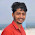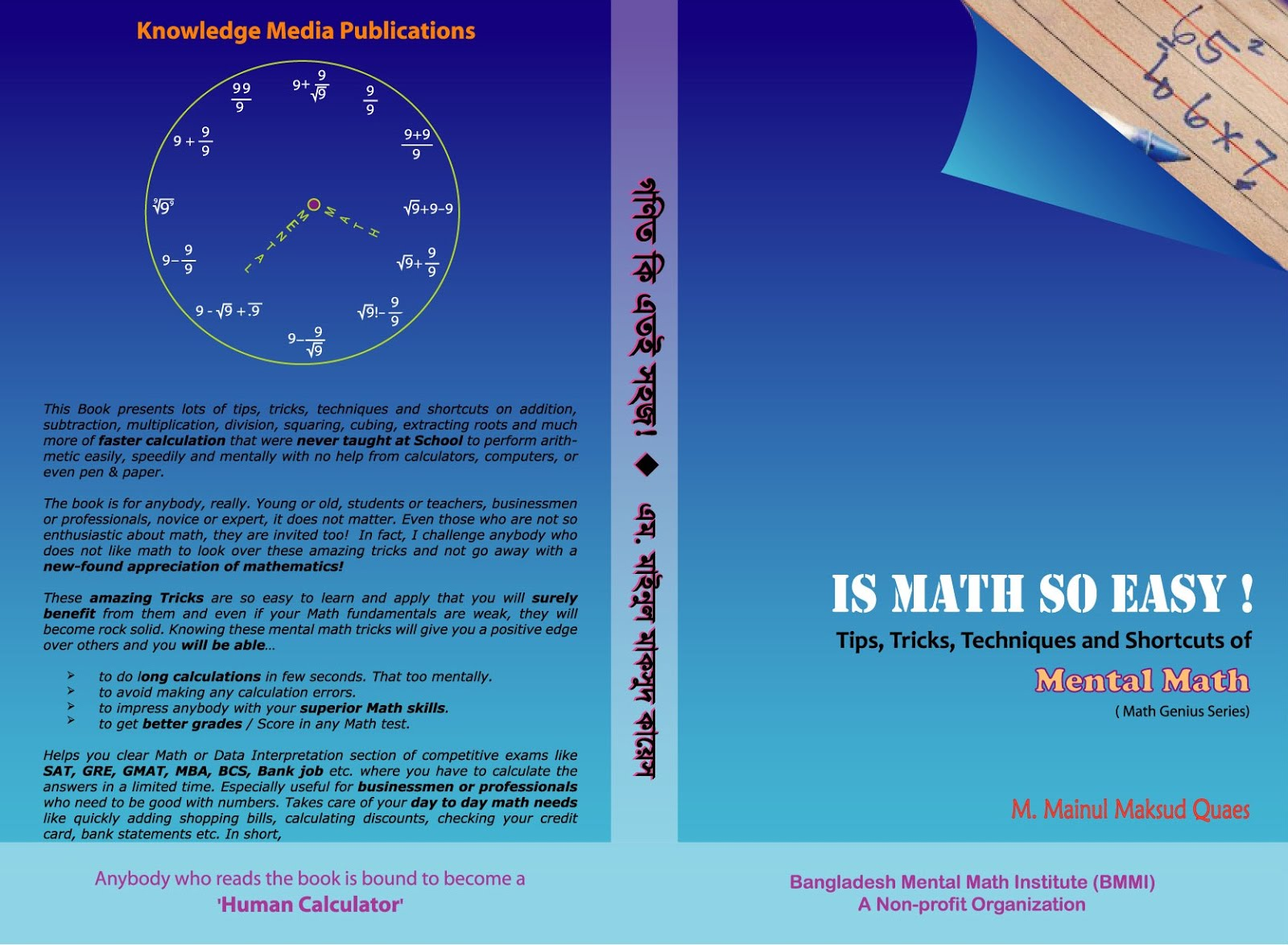# Easy trick to mentally calculate percentages

Situation when we need to calculate the percentages in our mind are quite common. While calculating the VAT, Insurance premium or Tips at restaurant, we thought of percentages or perhaps if we are earning then we would have thought about percentage of our salary we are paying for rent, food or fuel for our vehicle. These are real life situation and we are often asked in the competitive examinations.

Basic Percentage Review

So what is the easiest way to calculate percentages? Here are some tips to quickly find percentages mentally. Think like this:

• There are some percentages that are easy to calculate mentally. 50% means half, 25% means 1/4 and 75% means 3/4 .
• Finding 10% of a number is one of the most useful and also one of the easiest. To find 10% all we need to do is move/shift the decimal one place to the left. Thus 10% of 543 = 54.3, pretty simple!
• 1% is just as easy too. Shifting the decimal point to 2 digits left. So, 1% of 654 = 6.54
• 100% is the easiest by far. The number does not change. So, 100% of 765 is the number itself i.e. is 765.
• 50% is just the number divided by 2. So, 50% of 444 = 222.
• 15% is just 10%+5% and we already know how to solve these. So, 15% of 24 =? 10% of 24 is, well, take the decimal to one digit left to get 2.4 and 5% is just half of 10%. So we divide 2.4 by 2 to get 1.2. So, add them up 2.4+1.2= 3.6 which is 15% of 24.
• 20% of 120=? 20% is nothing but 10%+10% (breaking up into friendly parts). So, 10% of 120 is 12 (shifting the decimal one place to the left) and double of that would be 24. Again, 20% of 132=? 10% would be 13.2 and 20% would be double of that that is 26.4
• 60% of 320=? 60%=50%+10%, so 50% of 320 would be 160 and 10% would be 32. So, our final answer would be 160+32 which is 192.
• 75% is doing the same thing-breaking it up into friendly parts/numbers. 75% of 880=? You can break it up by 50%+25%. 50% of 880 gives us 440. Now we need to find 25% of 880. Now, 25% is just half of 50% and we already solved 50%, so just take half of that. 440 divided by 2 gives us 220. Now as we broke it up, we have to put it back together. So our final answer is 440+220 =660.
• 95% of 2400 =? Break it up into friendly parts/numbers. So break it 2 parts- 100% and 5%. The 100% of 2400 does not change. So, 100% of 2400=2400 and 5% of 2400=? To find 5%, take 10% and then divide by 2. 10% of 2400 is 240 and half of 240 is 120, so 5% of 2400 is 120. Now as we broke it up, we have to put it back together. So, 100% - 5% =2400-120=2280.
• 110% of 140=? 110%=100%+10%, 100% is the number itself which is 140 and 10% of 140 is 14 so 140+14=154.
We can use same breaking up techniques to solve ANY percentage problem.

• 31% of 222=? Breaking up 31% by 10%+10%+10%+1%. Now, 10% of 222 just gives us 22.2. Because we have 3 sets of 10%, we need to multiply 22.2 by 3 to get 66.6 (10%+10%+10% is the same as 3x10%). Lastly we need to find 1% of 222 which gives us 2.22. So, our final answer is 66.6 +2.22 =68.82
• 21% of 150=? 21%=10%+10%+1%. Now 10% of 150 would be 15. So, 20% would be double of 15 that is 30 and1% of 150 would be 1.5. So final answer would be 30+1.5 which is 31.5
• 17% of 300=? Break 17% into 10% + 5% + 2%. Now calculate; 10% of 300 is 30, 5% of 300 is half of 10% (i.e. 30/2= 15) and 2% (2x1% of 300 = 2x3 = 6). So it all add up to 51.
• Let’s try 97% of 195. 97% is probably broken down easiest into 100%-3%. 1% of 195 =1.95. Now multiply it by 3 to get 5.85. So our final answer is 195-5.85=189.15
• 98% of 250=? 98% is 100%-2%. 100% of 250 is 250 and 1% would be 2.5 (decimal will shift 2 places towards the left). 2% would be 5. So our final answer would be 250-5 i.e. 245.
• 167% of 195=? One way to break it up is 100%+50%+10%+5%+2%. We know 100% of 195 is 195. 50% is just half of 195 which is 97.5. 10% of 195 is just 19.5, 5% is 9.75, 2% of 195 is 3.9. And finally we just add them all up to get, 195 + 97.5 + 19.5+9.75+3.9 = 325.65
• There are many different ways to look at a problem. Say, 42% of 366=? 2 different ways to solve the same problem. We can do this by taking (a) 4x10% + 2x1% or (b) we can also do it a different way by taking 50% and subtracting 8% i.e. 50% - 1%x8. Both are same, both give us the same answer.  a) 4x10% + 2x1%. 10% is 36.6 and 1% is 3.66. Now, 4x36.6=146.4 and 2x3.66=7.32; adding them up is 153.72. b) 50% - 1%x8. 50% is half of 366 which is 183 and 8% is 3.66x8 = 29.28; subtracting the two gives us 183-29.28=153.72, the same answer as before.
• 62% of 420 would be 50%+10%+2% which is 210 + 42 + 8.4 = 260.4
• 22% of 324 would be 10%+10%+2% i.e. 32.4×2 +3.24×2 = 64.8 + 6.48 = 71.28
• 16% of 42 would be 10%+5%+1% i.e. 4.2 + 2.1 + 0.42   = 6.72.

a% of b = b% of a

• It may not be immediately clear, but it’s true: a% of b = .01 × a × b, which is the same as b% of a (.01 × b × a).
• What’s 16% of 25? The same as 25% of 16: 4
• What’s 43% of 200? Same as 200% of 43: 86.

1.wow, great, it was enlightening for me! thank you so much!:)

2.Thanks very useful

3.I think theirs a little mistake of example 5% of 44. It should be 5% = 2.2 :-)

1.Oh! yes Musa. Thanks. Now rectified.

4.Nice also provide quicker way to solve both square and square root. !!

1.Thanks, Rahul. Yes, already posted. Plz check...

5.cool tricks... thnx a lot.

6.Thanks Mainul for a great Post

7.Interesting post.
Thanks

Moving calculator

8.Thank you:) nice explanation:)

9.Thank you, that was great. now I can solve percentage without fear.

10.How to calculate 771/4048 * 100 ???

11.amazing article

12.What percentage of 1,110

13.Amazing blog. One can check the information about gate made easy online test series at Online ICE GATE Institute.

14.15.This is so helpful , I can't believe i will be able to solve Percentage Off - head from now. My gratitude to who ever wrote this is so Inexplicable. Thanks a lot!

16.Thanks for sharing such wonderful nd easy methods

17.Is it just 1% that moves the decimal two times to the left?

18.19.Can you suggest mental trick for
5448/5675*100

20.Amazing explanation. Thanks for sharing your knowledge. God bless you

21.ITS QUITE USEFUL....

22.Thanks this is really easy rather than the teacher explains for 10 minutes straight I could literally learn it in 5 seconds!!! Thank you sooo much this will really help in my half - yearly exam! Thanks i love uuuuu!!!!

23.if 60% of A is 30% of B , then B is what % of A????//

1.24.Its very usefull.... thank u..😊

25.Too much useful. now all doubts has cleared. a big thanks to you

26.usefull, thanks.

27.Many many thanks... very enrichment of skill again .. won't stop my self to say thanks again . I go banana for TR his

28.thank you very much

29.Thanks very much

30.If 5 litres of water is evaporated from 25 litres of a solution containing 12% of salt. What is the percentage of salt in the remaining solution

31.If 5 litres of Water is evaporated from 25 litres of a solution containing 12% of salt. what is the percentage of salt in the remaining solution.

32.Nice blog.
Really, you are sharing great information from your blog that is very useful for me. Thank you so much.
VAT Calculator

33.Great!! Thankyou mainul...

34.OMG!! Its very easy wid these tricks.. Tons n tons of thanks mainul..

35.36.Useful page. Great information with good explanation

37.Thank u sir u cleared my doubt corectly.

38.Thank you so much for this post.

I'd also add : "75%: 100%-25". I believe is easier.

39.It's just great. A beautiful trick very helpful

40.Wow nice thanks

41.Really, you are sharing great information from your blog that is very useful for me. Thank you so much. IST Merit Calculator

42.Informative and quick Learning
Keep it up

43.44.Thank you so much! It really helped me 😊

45.46.47.